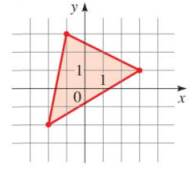Chapter 11.CR, Problem 71E### Algebra and Trigonometry (MindTap ...

4th Edition
James Stewart + 2 others
ISBN: 9781305071742

#### Solutions

Chapter
Section### Algebra and Trigonometry (MindTap ...

4th Edition
James Stewart + 2 others
ISBN: 9781305071742
Textbook Problem

# 7 1 − 7 2 . Area of a Triangle: Use the determinant formula for the area of a triangle to find the area of a triangle to find the area of a triangle in the figure.To determine

To find:

Area of the triangle by using the determinant formula.

Explanation

Given:

The figure of the triangle is,

Figure (1)

Approach:

If a triangle in the coordinate plane has the vertices (a1,b1), (a2,b2), and (a3,b3), then the area of the triangle is given by

A=±12|a1b11a2b21a3b31|

where the sign is chosen to make the area positive.

Calculation:

Figure (2)

As seen in Figure 1, the coordinates of vertices of the triangle are (1,3), (3,1), and (2,2)

### Still sussing out bartleby?

Check out a sample textbook solution.

See a sample solution

#### The Solution to Your Study Problems

Bartleby provides explanations to thousands of textbook problems written by our experts, many with advanced degrees!

Get Started